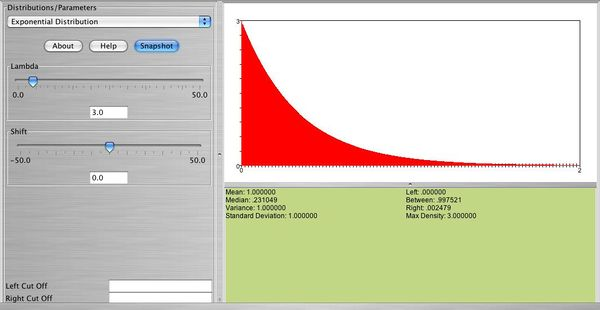# SOCR EduMaterials Activities Exponential Distribution

## This is an activity to explore the Exponential Distribution: Learn how to compute probabilities, densities, and percentiles.

• Here is the shape of the exponential distribution (this is a snpashot from the SOCR website):• Exercise 1: Graph and print:
• a. exp(0.2)
• b. exp(1)
• c. exp(10)
• Exercise 2: Locate the maximum density for each one of these distributions.
• Exercise 3: Find the height of the density at 3 values of X (one near 0, one near the mean, and one towards the tail of the distribution).
• Exercise 4: Find one percentile for each of these distributions and record them on the printouts. Verify these percentiles using the formula we discussed in class$x_p=\frac{ln(1-\frac{p}{100})}{-\lambda}$
• Exercise 5: Compute one cumulative probability for each one of these distributions, show it on the graph, and verify it with the formula$P(X \le x)=1-e^{-\lambda x}$